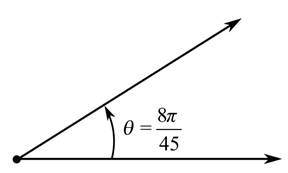Chapter 8.1, Problem 10E### Calculus: An Applied Approach (Min...

10th Edition
Ron Larson
ISBN: 9781305860919

#### Solutions

Chapter
Section### Calculus: An Applied Approach (Min...

10th Edition
Ron Larson
ISBN: 9781305860919
Textbook Problem
1 views

# Finding Conterminal Angles In Exercise 7-10, determine two conterminal angles in radian measure (one positive and one negative) for the angle.To determine

To calculate: The two coterminal angles in radian measure, in which one is positive and another is negative with the help of provided diagram,Explanation

Given Information:

The provided angle is θ=8π45 radians, which is shown below as,

Formula used:

The angle measuring θ has same initial and terminal sides as the angle measuring 360θ, such angles are coterminal angle.

Calculation:

Draw diagram for coterminal angles and provided angle for θ=8π45 radians.

From above diagram, let θ+ be the positive coterminal angle and θ be the negative coterminal angle

### Still sussing out bartleby?

Check out a sample textbook solution.

See a sample solution

#### The Solution to Your Study Problems

Bartleby provides explanations to thousands of textbook problems written by our experts, many with advanced degrees!

Get Started

#### In Exercises 7-12, refer to the following figure. 9. Which points have negative y-coordinates?

Applied Calculus for the Managerial, Life, and Social Sciences: A Brief Approach

#### Which property of real numbers is illustrated in each part of problem 3-6? 6. a) b)

Mathematical Applications for the Management, Life, and Social Sciences

#### Evaluate the definite integral. 01cos(t/2)dt

Single Variable Calculus: Early Transcendentals

#### f(x) = (x2 3)2 has points of inflection at x = _____. a) 0,3,3 b) 1, 1 c) 0, 1, 1 d) 3,3

Study Guide for Stewart's Single Variable Calculus: Early Transcendentals, 8th

#### Define the concept of external validity and a threat to external validity.

Research Methods for the Behavioral Sciences (MindTap Course List)